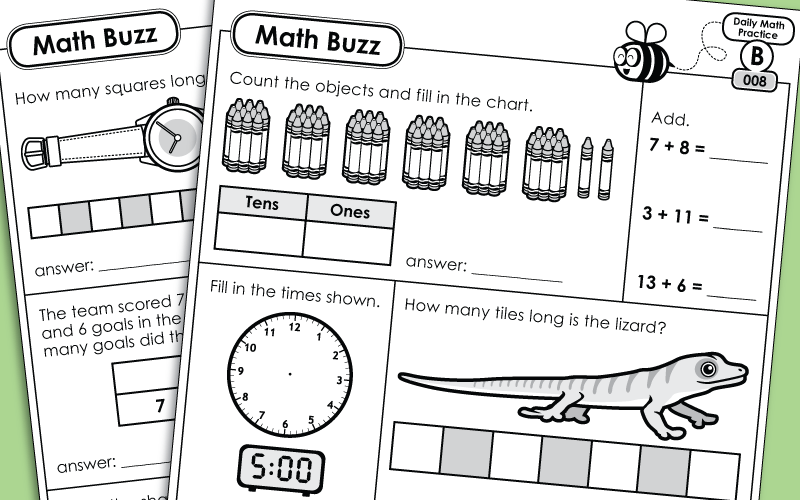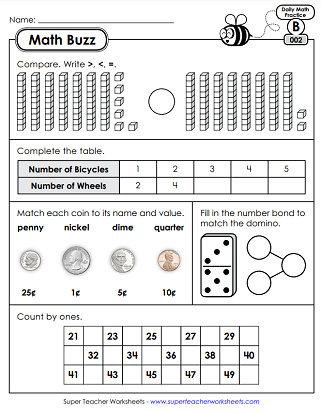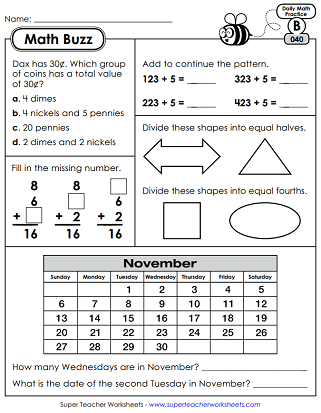# Daily Math Review Worksheets - Level B (2nd Grade)

You students will build and retain their understanding of important math skills with these daily, spiraled review worksheets. There are 150 worksheets in the 2nd grade Math Buzz set.This file has 5 worksheets, one for each day of the week. Skills include basic addition, place value with base-10 blocks, measurement with non-standard units, and solving word problems.
In this set, students will review skip counting by 5s, measuring with non-standard units, telling time to the nearest half hour, and comparing 2-digit numbers.
In week 3, students will review in-out boxes, addition, counting money, measurement with non-standard units, and part-part-whole models.
In the 4th week, students will practice attributes of solid shapes, value of the underlined digit, estimating length, and double-digit addition with regrouping.
In this set, kids will practice skip counting by 100s, solving word problems, dividing shapes in half, reading a line plot, and telling time (half hour increments) on an analog clock.
Use mental math to subtract a 1-digit number from a 2-digit number, find the value of coins, read and interpret a tally chart, measure to the nearest inch, and identify shapes that are divided into quarters.
On these daily review worksheets, students will review time (am/pm), add 2-digit numbers using the standard algorithm, solve word problems using part-part-whole models, identify flat surfaces on solid shapes, and answer questions related to place value (3-digits).
Add and subtract 2-digit numbers, choose the correct operations to solve word problems, compare 2-digit numbers, identify odd/even numbers, and match ordinal numbers with their word names.
In Math Buzz Week 9, your students will practice addition using the standard algorithm, identify numbers shown by place value blocks, count coins, and find missing shapes in patterns.
Use bar models to help solve addition and subtraction problems, differentiating between even and odd numbers, add using the standard algorithm, count money, and arranging objects in order of length.
Learn about halves, thirds, and quarters. Compare using the symbols for greater than, less than, and equal to. Use a centimeter ruler to draw lines. Add and subtract ten using mental math. Read times on analog clocks.
Use an inch ruler to draw lines. Express numbers in expanded and word form. Determine which measuring tool to use.
On these five Math Buzz worksheets, students will identify attributes of polygons, count coins, skip count by 5s, identify lines of symmetry, tell time to the nearest 5 minutes, and add three addends together.
Write money amounts using the fewest possible coins. Add and subtract using the standard algorithm. Write the values of the underlined digits. Use mental math to subtract 10 and 100. Divide shapes into equal parts.
Write the times shown on the analog clocks. Draw the correct temperature on the thermometer. Find days on a calendar. Read and interpret data on a line plot.
Tell how many vertices and sides a polygon has. Divide shapes into halves and quarters. Answer questions about a line plot. Subtract double-digit numbers using the standard algorithm.
Find the values of coin sets. Count backwards by 1s. Follow the rule to complete an input/output table. Estimate lengths. Solve problems related to arrays.
On these 2nd grade Daily Review worksheets, your students will review double-digit subtraction, counting money, writing times on analog clocks, basic multiplication with an array, and counting angles on shapes.
In MB #91 through 95, your class will practice 2-digit subtraction, counting money, 3-digit addition, solving word problems, and linear measurement.
On these worksheets, kids will find the answers to word problems, count month, complete input/output tables, find the sums for addition problems with 3 addends, and order numbers from greatest to least.
This week, students will match 3-digit numbers to their word names, add triple-digit numbers, tell whether numbers are even or odd, count money, compare numbers, and practice repeated addition.
Estimate lengths of animals. Solve addition and subtraction word problems. Determine if a number is even or odd. Use a running total to count money. Complete a bar graph.
In the 23rd week of 2nd grade Math Buzz, students will divide objects into equal fractional parts (halves), create a shape with 3 angles, subtract a single-digit number from a 3-digit number, read and interpret a line plot, and compare money amounts.
In the 24th week of Math Buzz, students will add and subtract 3-digit numbers, count angles in a polygon, estimate lengths, read and interpret a line plot, and divide a rectangle into an array.
This week student will: identify basic shapes, add and subtract triple-digit numbers, count money, compare numbers, and use arrays to solve repeated addition problems.
On these worksheets, pupils will: count backwards by 1s, differentiate between triangles and quadrilaterals, find sums and differences, answer questions about a calendar, and solve an elapsed time problem.
Review and practice making a line plot, finding sums and differences, ordering numbers from least to greatest, measuring to the nearest inch, and counting halves.
Complete a line plot using these data shown. Count backwards by 100s. Solve an addition problem with multiple addends. Plot points on a number line. Tell how many thirds make a whole. Measure lines in centimeters.
Find the elapsed time. Subtract 2-digit numbers. Count by 10s. Calculate change. Use a bar model to solve a problem. Complete a bar graph.
In the 30th and final week of 2nd Grade Math Buzz, students will: solve an addition problem with 4 addends, shade an array to solve a repeated addition problem, complete a picture graph, count money, and plot numbers on a number line.
More Daily Review (Math Buzz)

Are these problems too easy or too hard? Would you like to try a different grade level of Math Buzz? Check out the full Daily Math Review index page for a complete list of all levels available.

## Sample Worksheet ImagesMy Account
Site Information